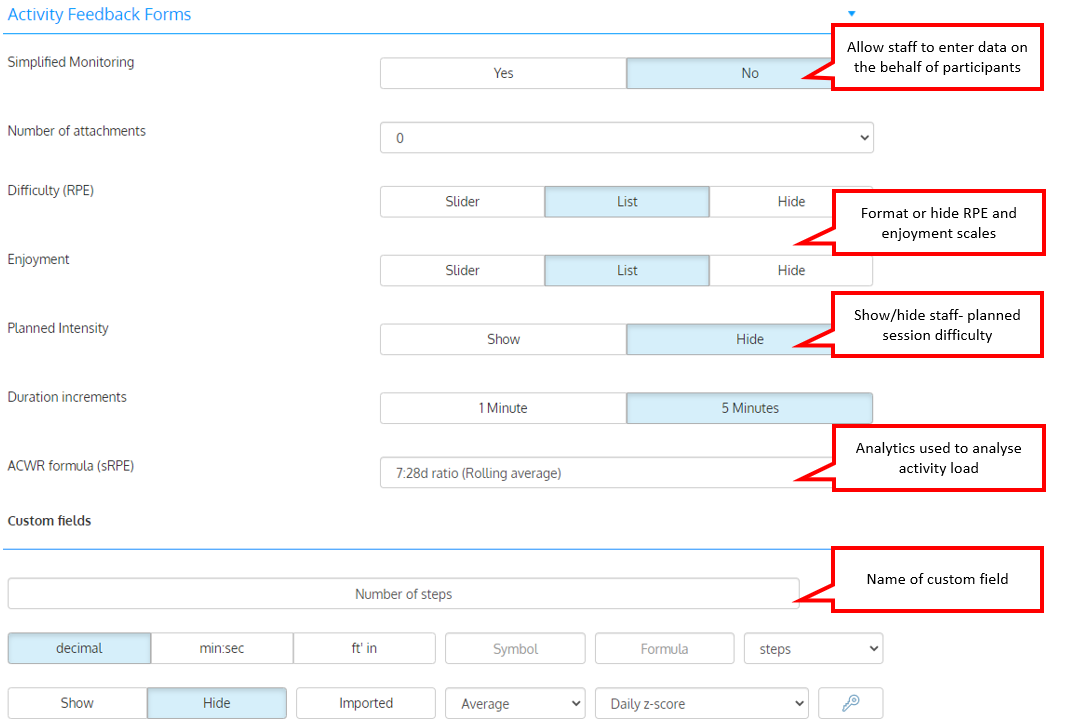You can configure the participants' data collection form extensively and add up to 150 custom fields to collect additional information. Each field can be configured at will and activated /deactivated at any time.

Setting up the training data collection formsConfiguring custom fields

Using formulas to calculate custom fields

You can use your own formulas to combine multiple fields into a single measure and calculate your own custom metrics.

For example, you can create a 'session stress index' by dividing the RPE by the session's duration, or calculate a 'Total distance' from different activities performed during a session (example below).

To use a custom field in a formula,  enter its corresponding symbol (string of 6-10 lowercase letters of your choice) in the Symbol box.

To create a formula,  enter the corresponding combination of symbols, constants, operators and functions in the Formula box.

Symbols should only contain lowercase letters (eg: a symbol such as "20mSprint" will not work but "twmsprint" will). Symbols containing spaces, numbers or uppercase letters will not work. Do not add a '=' sign before the formula, like you would do in Excel.

Formulas are automatically processed when sessions are saved.

The section below presents the operators, functions, constants and variables that you can use in formulas and provides you with examples.

Variables you may use in formulas

age = age calculated using the birth date, according to the information stored in the participant's profile
gender = sex (M or F)

Any symbol representing other custom fields ('rundist' =running distance in the example above)

Math operators

- (substraction)
/(division)
* (multiplication)
^ (power)
() parenthesis

Logical operators

> (greater than)
< (less than)
<= (less than or equal)
>= (greater than or equal)
== (equal)

Built-in variables

age= calculated using the athlete birth date, according to the information stored in the athlete profile
gender=gender according to the information stored in the athlete profile (M/F)

duration=duration of the session

rpe=participant-selected RPE/difficulty of the session

enjoyment=enjoyment recorded for the session
Any symbol associated with another custom field

Built-in mathematical functions

FITSTATS use a powerful mathematical library that includes many functions that you can use in formulas. For a complete list, click here . All functions listed can be used in FITSTATS  formulas.

For example, you can use a number to the 2nd decimal with the formula : round(symbolone/symboltwo,2)